# Counting American Money with Examples

Counting American Money with Examples• We want to find the total amount of money shown.
• We have a dollar bill, three dimes, a nickel and two penny coins.
• The dollar bill is worth 100 cents.
• Each dime is worth 10 cents, so three dimes are 30 cents.
• The nickel is worth 5 cents.
• The two penny coins are worth 2 cents in total.
• We add the total using column addition to get 137 cents.
• We can write this in dollars as $1.37 by dividing 137 by 100 We add the cents up and convert the value to dollars by dividing by 100. We write our answer with a dollar sign, ‘$’ and no longer write the cent sign, ‘¢’.• We will count the amount of money shown.
• We have one dollar bill, two dimes and four nickels.
• The dollar bill is worth 100 cents.
• The two dimes are worth 20 cents because 2 x 10 = 20.
• We can add the dollar and the two dimes to make 120 cents.
• The four nickles are worth 20 cents because 4 x 5 = 20.
• We add this 20 cents to our existing 120 cents to make 140 cents in total.
• We divide 140 cents by 100 to convert our value in cents to a value in dollars.
• We have $1.40.#### Counting Money: Interactive Question Generator #### Counting Money: Interactive Question Generator #### Counting US Money Worksheets and Answers #### Counting Money with Regrouping Worksheets and Answers ## Adding American Money To add money, write the values of each coin and then add the numbers using vertical addition. It is useful to collect the coins into groups that form a multiple of ten cents or a dollar. In this lesson, we learn how to add dollar bills and count cent coins to make a total. In the example below, we have some American money. We will add the values of the cent coins to the value of the dollar bill to see how much money there is in total. We can do this by first adding up our total entirely in cents.There is a$1 bill, which is worth 100¢.

The dollar is larger in value than the American cent coins and so we write this 100 cents first.

We then have three one dime coins (each worth 10¢), one nickel (worth 5¢) and two penny coins (each worth 1¢).

We can add the ‘ones’ column:

0 + 0 + 5 + 1 + 1 = 7.

We now add the ‘tens’ column:

0 + 3 = 3.

In the hundreds column, we only have 1.

Therefore our total value of money is 137¢.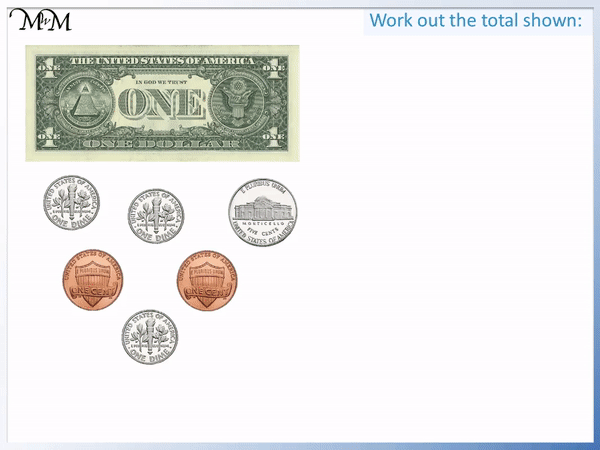If the total amount of money is more than 100 cents in value, we usually write this amount of money in terms of how many dollars we have. We will convert our total cents into dollars.

There are one hundred cents in a US dollar.

To convert from cents to dollars, we divide by 100.

137 ÷ 100 = 1.37

We write our total as $1.37. We separate the dollars and the cents with a decimal point. So, we say we have one dollar and thirty-seven cents. You will notice that we only write the dollar sign, ‘$’ in front of our total and if we choose to write our amount in dollars, we do not write the cent sign, ‘¢’ anymore.

We write how many dollars we have made in front of the decimal and then the two digits that come after the decimal point represent how many more cents that we have.

Below, we have another example of counting US money: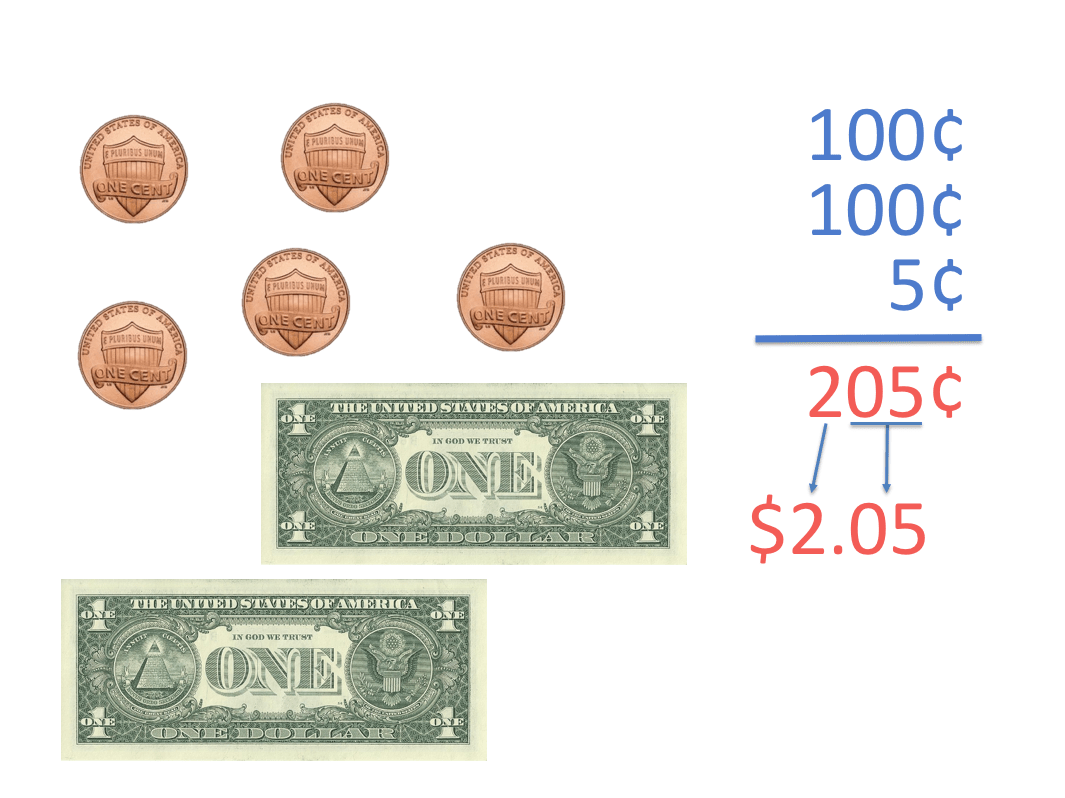There are two $1 notes, which are each worth 100¢. There are also five 1¢ coins, which are worth 5¢ in total. We write the largest values of money first. Using column addition, we will add up the total of our money. In the ‘units’ column of our cents we have: 0 + 0 + 5 = 5 We have no ‘tens’. Counting the ‘hundreds’ column, we have: 1 + 1 = 2 In total, the amount of money is 205 cents. Remember that we usually write our amount of money in terms of dollars if we have more than 100 cents in total.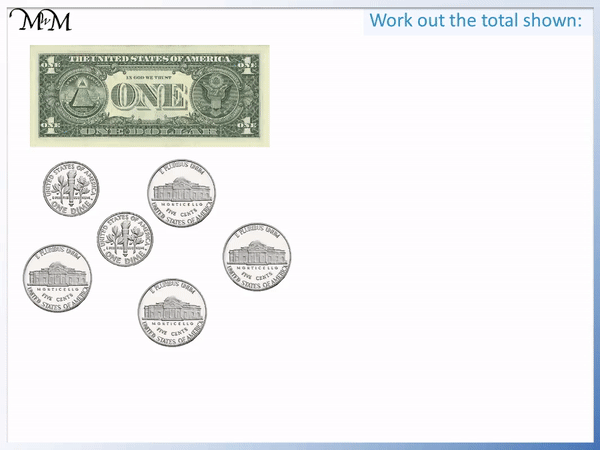There are one hundred cents in a US dollar, so to convert our cents to dollars, we divide the amount by 100. 205 ÷ 100 = 2.05 So we can write our total as$2.05.

We can say that we have two dollars and five cents.

When converting our cents to dollars and then writing our answer in dollars, we always write two digits after the decimal point. However, the five cents is only one digit.

Therefore, we must keep the zero in front of the five to ensure that there are two digits after the decimal point.

And so, we write this as $2.05. We separate the dollars and the cents with a decimal point. ## Adding Dollars and Cents To add amounts of money that contain both dollars and cents, it is helpful to count the dollars separately to the cents and add them at the end. It is also useful to count the cents in groups that make multiples of ten cents or a dollar. Add the larger value coins first. To help count the cent coins, it is useful to know the groups of coins which make a dollar.The dollar bill is worth 100 cents. The following amounts of money can be replaced with a dollar: • 100 penny coins • 10 dimes • 20 nickels • 4 quarters Any combination of coins that make 100 cents can be replaced with one dollar. Here is an example of adding a sum of money involving dollars and cents.To add this sum of money, separate it into dollars and cents. There are 2 dollar bills and the rest are coins. We look for groups of coins that add to make a dollar. We have ten dimes. We can move these across to the dollars pile to make$3 in total.

We have a dime and a penny left over. This is worth 11 cents.

There is $3.11 in total. When teaching children to add money, it is helpful to count the dollars and cents separately. This provides them with a strategy to use to break down the process. In the example below, there is some American money. We are asked to work out how much money there is in total. We will start by separating the money into dollars and cents.We can now add the money by first counting the dollars and then counting the cents.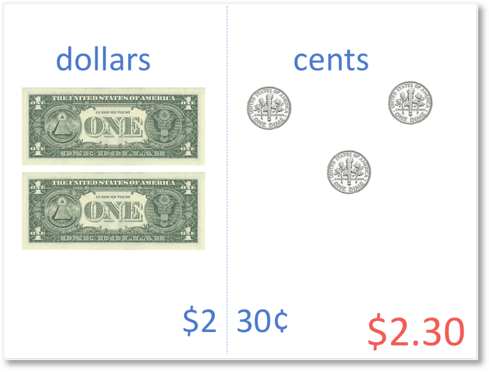Looking at the dollars on the left, we can see that there are two one dollar bills. So, this is worth a total of$2.

Looking at the cent coins that we have, we can see that there are three dimes. Each dime is worth 10¢. So, this is worth a total of 30¢.

Altogether, we have two dollars and thirty cents.

We write this as $2.30, separating the dollars and cents with a decimal point. We can think of the digit before the decimal representing how many dollars we have and then the two digits after the decimal representing how many cents we have. In some cases, there will be a group of cent coins that can make another dollar.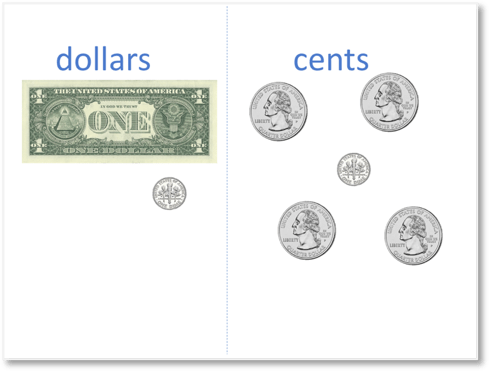We will begin by separating the US money into dollar bills and cent coins.Now we have separated the paper notes from the coins, we will count the dollar bills. There is a one dollar bill, so we have$1 in total from the paper bills.

Next, we will count the cent coins.

Remember that we are looking out for any of the combinations of coins that can add up to make a dollar.

We can make a dollar from any combination of coins that add up to 100 cents.

A tip for teaching this topic of counting money is to look for the largest value coins first as they will help us to reach 100 cents faster.The cent coin with the largest value is the quarter.

Looking at this group of cent coins, we can see that we have four of these quarter dollar coins.

These four quarters make a total of one dollar.

This is because they are each worth 25¢ and

4 x 25¢ = 100¢.

We can move these four coins over to the dollars. We now have $2; one dollar from the paper bill and one dollar made from the four quarter dollar coins. We can say that we have collected the four quarters into a group of one dollar. When teaching this in schools, this process may be referred to as ‘regrouping’ or ‘carrying’, since we have regrouped 100 cents into 1 dollar. Finally, we’ll look at the cent coins that remain.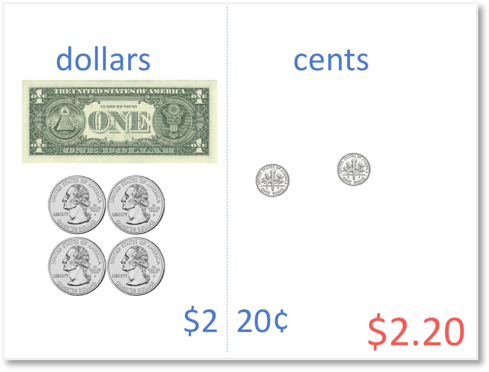Looking at the cents, we can see that we have two dimes remaining. Each dime is worth 10¢. So, two dimes are worth a total of 20¢. Altogether, we have two dollars and twenty cents. We write this total as$2.20.

Remember that the ‘2’ in front of the decimal point tells us the total amount of dollars that we have made and the ’20’ after the decimal point tells us that we have twenty cents on top of this.

Below, is our final example of adding money with regrouping.Again, we begin by separating the money into paper dollar bills and cent coins.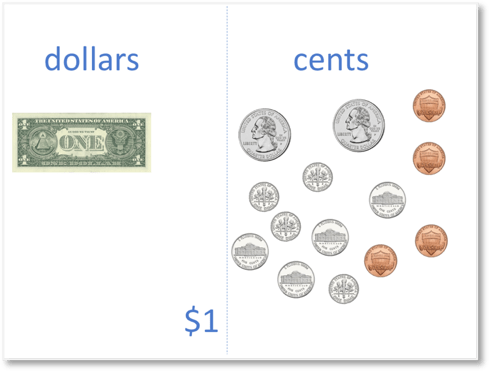Looking at the dollars, we can see that we have a one dollar note. This is a total value of $1. Next, we’ll look to see if we can make another dollar out of any of the coins that we have. This means that we need to make a total of 100 cents by adding up the coins. Remember that when teaching this topic, we encourage you to look for the coins with the largest value first as they bring us closer to 100 cents in the fastest way.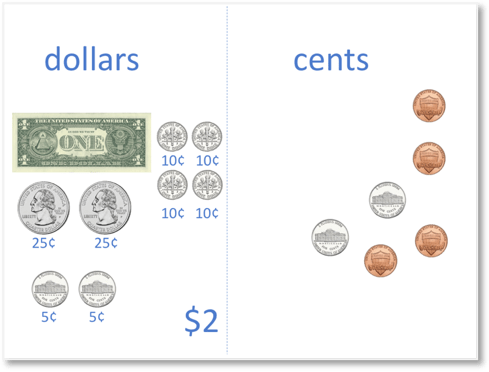Our largest value cent coin is the quarter. There are two quarter dollars, each worth 25¢. 25¢ + 25¢ = 50¢ So far we have 50¢. Our next largest value coin is the dime, so we now look to move the dimes over until we get to 100 cents or until we run out of dimes. We have four dimes in total. Each dime is worth 10¢, so four dimes are worth a total of 40¢. 50¢ + 40¢ = 90¢ So far we have 90¢. We need 10¢ more. The next largest value coins are nickels, which are each worth 5¢. So, two nickels are worth a total of 10¢. 90¢ + 10¢ = 100¢ 100¢ =$1

Having moved these two nickels over, we have a total of 100¢. We only moved two nickels over, we left another two nickels behind as we made 100 cents without them.

There are 100¢ in a dollar. So, in total, we now have $2. One dollar from the paper dollar bill and one dollar from the collection of different coins that made 100 cents. Now, we will count the cents that are remaining on the right.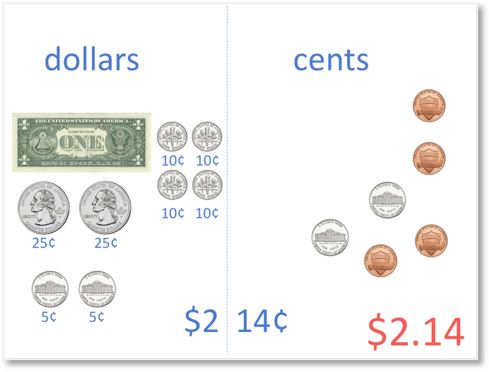There are two nickels which are each worth 5¢. 5¢ + 5¢ = 10¢ Two nickels are worth 10¢ in total. We also have four pennies. These are each worth 1¢. 4 x 1¢ = 4¢ Four pennies are worth 4¢ in total. Two nickels plus four one cent coins make a total of 14¢. 10¢ + 4¢ = 14¢ Altogether, we have two dollars and fourteen cents. We write this as$2.14.Now try our lesson on Adding US Money by Regrouping Cents to Dollars where we learn how to regroup cents to make a dollar and use this to work out larger totals of money.

error: Content is protected !!